## FANDOM

10,619 Pages

•## How do indescribables work in OCFs?

July 23, 2019 by Ubersketch

Specifically talking about OCFs by Rathjen and Stegert.

•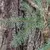## Can someone teach me how Rathjen’s OCF works?

July 23, 2019 by SuperSpruce

I really want to know how higher OCFs work and I don’t want to fall into the UNOCF trap. Could someone walk me through, step-by-step, of how Rathjen’s OCF works? Note that I know Madore’s Psi and I use extended Madore’s Psi in comparisons.

•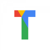## HashNo

July 22, 2019 by TGReddy

Introducing HashNo, my latest attempt at a decent notation.

https://tgreddy.github.io/main/

- - - - -

Can someone help me analyse my functions?

•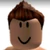## Rubiks numbers

July 22, 2019 by Angrybirdstd

Rubiks numbers are combination counts of rubiks cubes. The numbers are represented as RUBIKS with a subscript saying length of one side(dimensions). RUBIKS 2(3) is the combination count of a cube with a height of 2 cells and in 3 dimensions. RUBIKS 1000(11) is the combination count of a hypercube with a height of 1000 cells and in 11 dimensions. That is a LOT.

GUBIKS number or RUBIKS 10(100).

GUBIKPLEX number or RUBIKS 10(RUBIKS 10(100)).

MegaRUBIKS or RUBIKS 1000000(1000000).

•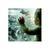July 22, 2019 by Scorcher007

Hey. Does anyone have any ideas how to simplify talk about the Cofinality with person who has just begun study the ordinals? Perhaps some analogies or examples not related to a strict formal definition, such as "mappings function", etc....

•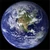## Pooping in a pool

July 21, 2019 by Pi.jayk

Have you ever pooped in a swimming pool

•## Who is Pi.jayk exactly?

July 21, 2019 by Ubersketch

Something happened to this wiki recently.

A user named Pi.jayk has requested for this wiki to be deleted. Though it's unlikely this request will be fulfilled (it's probably made to scare people and cause controversy, and this wiki is very active), I have reasons to be concerned about this.

https://googology.wikia.org/wiki/User:Pi.jayk

The link to his Facebook is broken, but we have a discord tag: Pi.jayk#1725

Nothing about else his Googology userpage stands out so I'll look at his contributions instead.

https://googology.wikia.org/wiki/User:Plain%27N%27Simple?oldid=262445

Now looking at his Discord, he's on the Googology Discord (and on t…

•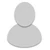## Blog post: 1-D Line notation

July 21, 2019 by Bogush4

Well, I have been interested in googolology for months, and I want to show my new notation... 1-D Line notation!

Basically 1-D Line notation is a notation that can be converted to up arrows. Here is the steps to convert

1. (10,100,2)=(10^100,1)
2. (10^100,1)=10^^100=giggol
3. Giggol=10^………^10^10

1. Erase any ones. (1,10,100,1)=(1,10,100)=(10,100)=10^100
2. If last=0, do multiplication. (10,100,0)=10*100=1,000
3. And also, if second last one = 0, then do not include it (2,10,0,1)=(2,10,1)=10^10=10,000,000,000
4. And if first is 0, then no base (0,10,100,1)=100

Well, the first is the replicant, then the base, then the determent, then the number of up arrows (R,B,D,Up).

Googol=(10,100)

Googolplex=(2,10,100)

•## Closing the wiki

July 20, 2019 by Pi.jayk

I requested FANDOM to delete this wiki

•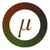## Everlasting Egrets

July 20, 2019 by QuasarBooster

Self-modifying bitwise cyclic tag (SBCT) is a simplification of the Turing complete esoteric language, bitwise cyclic tag, where the program string also functions as its own data string. The following is a summary of the computation rules, referenced from the language's page.

An SBCT program is any finite string of bits (commands), executed as follows:

• 0: Delete the leftmost bit.
• 1: Goto the next command (say x). If the leftmost bit is 1, copy x to the right end of the string.

If the program-string is initially empty, execution halts immediately; otherwise, starting at the leftmost bit and halting only when the string becomes empty, the commands are executed in cyclic sequence from left to right (the leftmost bit following next after the right…

•## Letter notation extension to Q (e0)

July 17, 2019 by Bubby3

I am going to extend PsiCubed's letter notation up to epsilon-0, with Q, before he makes an offical extension.

With the definitoin of the array notation and the ltter P, one can easily define palanar array notation function. In this notation, the comma is short for , and the dimensional seperator is . Z's represent 0's and dimensional seperators (or can be empty), and k zeroes. k+1 zerores means a group of zeroes and dimensional seperators starting with at least 1 zero. k zeroes is that but it doesn't need to start with a zero

1. Other rules are the same from linear array notation
2. (a,b,c...m+10,z)|x = (a,b,c,...,m1,0,0,z.)|10^(x-1) for 1=1 or there is only 1  and there is a 1 before it.
1. = (a,b,c,...,int(x)frac(k),z)| otherwise
3. (.…

•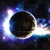## Busy Beaver Self Bitwise Cyclic Tag

July 16, 2019 by MilkyWay90

This will be a Busy Beaver but for Self Bitwise Cyclic Tag.

$$SBCT(n) =$$ the largest length of a terminating program of length n in SBCT

$$SBCT(0) = 0$$ the empty program terminates immediately

$$SBCT(1) = 1$$ the program 0 terminates after 1 iteration

$$SBCT(2) = 3$$ the program 10

•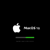## Generalized system for -illion-like and -yllion like suffixes

July 16, 2019 by Nirvana Supermind

Most people reading this should know that an n-illion is 103(n+1) assuming latin prefixes. A 100 ”cent”-illion is 10^3(101) or 10^303, a 2”bi”-illion is 10^3(3) or 10^9, and so on. This -illion suffix is used in many large numbers today. A -yriad suffix represnting 102(n+1) also started being developed from an unkown source and it can be found on fakes of this wiki today.

Both of these suffixes share one thing in common: they are of the form 10x(n+1), where x is an integer assuming n is replaced with a latin prefix for n. Ηοwever this is no coincedence. This general formula applies to the suffixes -illion, -yriad, -illiob, and several more with the values of x in each affix being 3, 2, and 10^3n+3 respectively. The formula also very closely m…

•## What's wrong with my code?

July 15, 2019 by QuasarBooster

Edit: This issue has been resolved so this post is no longer relevant. I'll keep it up for posterity though.

I recently discovered self-modifying bitwise cyclic tag and started making a program that stimulates its behavior. ) given on the language's page, as well as for smaller examples as far as I can tell. The issue is that it seems to mess up in the long-term for the given example. On the page it says the program terminates in 43074 steps but it doesn't seem to terminate with my code. Since more steps weren't provided for me to check against, I can't tell how I made a mistake. Could somebody please point out where I'm going wrong if they see it?

•## Growth rate of oracle Turing machines

July 14, 2019 by Ecl1psed276

It is known that the Busy Beaver function BB(n) outgrows all recursive functions, so we could say that it has a growth rate of $$f_{\omega_1^{CK}}$$ in the FGH, for a suitable system of fundamental sequences below $$\omega_1^{CK}$$ (Kleene's O may be used to do this).

However, consider if we make a special type of Turing machine, called the Oracle Turing Machine (OTM), that has another special state in addition to the "halt" state. This new state is called the "oracle" state. If the turing machine is put into this state, then we let N be the total number of 1's currently on the tape. We then determine whether Turing Machine #N terminates, given a well-ordered enumeration of all (non-oracle) Turing machines. (It doesn't matter exactly how th…

•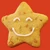July 14, 2019 by DrCocktor

He hasn’t edited since apr 2

•## Bitwise Cyclic Tag

July 13, 2019 by QuasarBooster

I've been exploring a few computational models recently. I ran into this variant of tag systems and cyclic tag called "bitwise cyclic tag". It's an incredibly simple esoteric language that's Turing complete! You can read about how it works in the link below.

I have an uncomputable function in mind that involves BCT and I was wondering if it would be valid to make a new page for it on the wiki. It would fit right in with the busy beaver and Xi functions. I could reference this link which directly mentions the author. Would there be anything wrong with making a page regarding this function and BCT?

https://esolangs.org/wiki/Bitwise_Cyclic_Tag

•## Googology Slack Server

July 13, 2019 by DrCocktor

I came here to do googology. I did not come here to be inappropriate. While I was blocked, I created a googology Slack server. http://googology.slack.com Send me your emails in the comments section so I can invite you guys.

•## Reminder to join the discord server

July 12, 2019 by Ubersketch

https://discord.gg/HYCeKZ4

•## An outline for an ordinal notation

July 11, 2019 by Ubersketch

I will be introducing Ubersketch Worm, USW for short.

1. ()=0
2. (#,0)=(#)+1 where # is any string
3. n+1 finds the leftmost n before n+1 if one exists, and replicates it infinitely (w times) and then gets killed
4. n+1 turns into n if the leftmost n before n+1 doesn't exist.

Evaluation goes from right to left.

empty string

•## DerivedSugar117 strikes again.

July 11, 2019 by Ubersketch

Recently a user by the name of cruckycrudge#8389 came into the Discord server, spat out a bunch of slurs, and linked us to this:

https://googology1.fandom.com/wiki/Googology_Wiki

Apparently, they want to make an alternative Googology Wiki, with the front page copied and pasted into here. One of the interesting things I noticed is that there was a "Big news" section where it says "Jonathan Bowers plans on creating videos about his arrays" which seems strange as that is the only piece of text there. There's a pretty big chance that this is by DerivedSugar117 so I decided to look deeper. There's only one user.

https://googology1.fandom.com/wiki/Special:ListUsers

Not DerivedSugar117. It seems likely that DerivedSugar117 used a sockpuppet to build …

•## Why was my blog deleted and by whom?

July 11, 2019 by Mh314159

Edited -- I misunderstood the actions of the moderator; it was not my blog or comment that was deleted.  The formatting of the deletion log was a bit confusing to me.  Is there a way for a user to delete his own blog post or to at least edit the title?  I cannot find the function for doing that.

•## Finishing my very first notation - The Generalized Factorial

July 10, 2019 by MilkyWay90

$$F$$ will be used to refer to any array notation where the exclamation mark is not in any valid expression in $$F$$.

$$F_{!}$$ will be used to refer to our superset version of $$F$$ with the factorial. Expressions in $$F_!$$ do NOT necessarily have a factorial.

$$s$$ will be used to refer to an array of natural numbers and factorials.

Define $$F_!(s)$$ in the following way:

1. If all elements of $$s$$ are natural numbers (without any factorials):
1. Set $$F_!(s) = F(s)$$.
2. If 1. is not true and that all entries of $$s$$ are $$1$$'s or exclamation marks is true:
1.  $$t =$$ the array obtained by replacing all exclamation marks by $$1$$.
2. Set $$F_!(s) = F(t)$$.
3. Otherwise, suppose that some entry of $$s$$ is a natural number greater than $$1$$.
1. $$s' =$$ the ar…
•## Useless harassment bot DerivedSugar

July 10, 2019 by Ubersketch

DerivedSugar has recently started harassing Pbot and other members of this community for no apparent reason and not listening to people's criticism. See for yourself. https://googology.wikia.org/wiki/User_blog:Mh314159/The_psycholog_of_large_numbers

https://googology.wikia.org/wiki/User_blog:DerivedSugar177/Doom_Function#comm-60626

https://googology.wikia.org/wiki/User_blog:P%E9%80%B2%E5%A4%A7%E5%A5%BD%E3%81%8Dbot/New_Googological_Ruler

https://googology.wikia.org/wiki/User_blog:P%E9%80%B2%E5%A4%A7%E5%A5%BD%E3%81%8Dbot/Elementary_Large_Number_beyond_PTO(%CE%A011-CA0)

https://googology.wikia.org/wiki/User_blog:P%E9%80%B2%E5%A4%A7%E5%A5%BD%E3%81%8Dbot/Ill-definedness_of_BIG_FOOT,_Little_Bigeddon,_and_Sasquatch

https://googology.wikia.org/wiki/Use…

•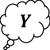## Another question

July 9, 2019 by Ynought

I had an idea for a function that possibly outgrows $$f_{\text{PTO}(\text{ZFC})}(n)$$ but if there exists an formal theory,lets call it $$\text{L}$$,such that $$\text{PTO}(L)\geq \omega_1^{\text{CK}}$$.Because if such an theory does exist then the function could be the either the fastest growing computable or one of the fastest growing uncomputable ones.Thanks for your help.

•## The psycholog of large numbers

July 8, 2019 by Mh314159

As a newcomer, I pause to wonder about the pyschology of big numbers.  Why do we and others find them compelling? My first fascinationg goes back to an essay by Asimov called "The Lore of Large Numbers.  And recently, I was drawn back to them after a discussion with some students about Steinhaus's Mega, and then Megiston, and then Knuth up-arrows, and then Graham's number, and we all were amazed that processes existed to make numbers that were unimaginably large (and even Mega qualifies as unimaginably large).  However, we were less fascinated by TREE3. We had some idea of what the tree drawing game was that defines it, but had no way to come to grips with a process that can compute it, and the fact that it is in fact large to an unknown d…

•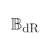## List of Common Failures in Googology

July 6, 2019 by P進大好きbot

I listed common failures appearing in definitions of large numbers. See also the list of common mistakes and questions on formal logic appearing in googology.

It is a common enemy to googologists. When you submit your blog post, then you will find many typos. When you extend your system, then you will find new typos. When you fix typos, then you will find that your correction generated other typos. The least weakly typo cardinal can be used to diagonalise higher inaccessible typos. It can be replaced by the least recursively typo ordinal so that it works in $$\textrm{ZFC}$$ set theory.

Hear ar exanples of tyops.

Definitions should be described in clear sentences without much ambiguity. Of course, sentences in a natural language usually includ…

•## new YIP notation

July 5, 2019 by Mh314159

This is my most current version of YIP notation so far, make the best use I can of the excellent  P進大好きbot's advice.  Thank you to everyone who looks at this and has feedback.

Rule set F

a, b are nonnegative integers

f0(a) = a + 1

fa(0) = fa-1(a)

fa(b) = fa-1fa(b-1)(fa(b-1))

Example: f1(2) = f04(4) because f1(1) = f0f1(0)(f1(0)) = f0f0(1)(f0(1)) = f02(2) = 4, so f1(2) = 8

Example: f1(3) = f08(8) = 16

so f1(x) = (2)(x+1)

Rule Set U

Universal Relationships

gp(x) = xgp-1(x)(x)

g0(x) = xx(x)

my(x) = my-1my(x-1)(x)

my(0) = my-1(y)

m0(x) = m-1m0(x-1)(x)

m0(0) = m-1m(m)

Rule set 1

One bracketed number

a, n are nonnegative integers

[a] = gnn(n)

if a = 1, n = 1

if a > 1, n = [a-1]

00(x) = fx(x)

Rule set 2

Many bracketed numbers

drop trailing zeroes

all entries contained in bracket…

•## YIP notation

July 4, 2019 by Mh314159

In a recent post I lamented that I am a dog, which unlike cats, cannot climb TREEs.  Actually, given how small my growth rates have been, I am a puppy.  And puppies go YIP.  So here is YIP notation.  I have tried to break my habit of thinking linearly.  So far, my notation only goes to [a,b] where (b+1) is the dimensionality of the recursion structure.  I'm not sure yet what [a,b,c] would mean, but probably something like recursing the dimensionality using dimensional numbers.  Anyway, before I go much farther, I'd love to know if what I'm doing is better than what I've done in the past or if it's just more of the same in a different format.  Thanks!!

↓x/indicates beginning and end of subscript

↑x\indicates beginning and end of superscript (…

•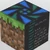## PEGG detailed log/N

July 4, 2019 by Naruyoko

You can see other PEGG-related things here.

Calculator can be found here.

(Update and calculation in progress...)

Starting Values:

• X=2.0000003409
• Y="N"
• Z=0.0005764801
• K=100000000000

date what number DJIA on
previous day ended with hundredth place of the
DJIA closing value (n) Z*n^2 X PEGG value (words representation)
M10.0377820263
M4JF62.412*10^63720660
M4K4J862.4385064920
M4L6J7G2F2E33.9*10^1514416621
M4(Lf)3(Le)2K2J2F3E3.684586382*10^21
M5(Le)4LK2JF72763.71402715
MeM5HfHeH2G72.539585396*10^17
(Me)6J2(Hg)3HeH8F7.8269436*10^1385
Mf(Me)2M2L2E7.588569339*10^21
Mf(Me)4M6LeL7K4J2E2503958886848829938660
(Mf)2JF2E1.1876*10^3616092
(Mf)2M6L6K2J6(Hh)6(Hg)4HfH6G3FE23.960*10^24187501
(Mf)3(Me)2M4K2J4H6E26.549629067*10^31
(Mf)3(Me)8(Lhf)8(Lhe)2(Lg)5LfJ4(Hh)3(Hg)4(Hf)2(He)7…

•## BEAF EXTENDED

July 3, 2019 by DerivedSugar177

Same rules as tetrational arrays

{(1)L}b,p = {LLL..LL}b,p.  With p Ls

{(2)L}b,p = now with {(1)L}b,p Ls

{(n)L}b,p = with {(n-1)L}b,p Ls

{(0,1)L}b,p = {(p)L}b,p

{(1,1)L}b,p = with {(0,1)L}b,p Ls

{(n,1)L}b,p = with {(n-1,1)L}b,p

{(0,2)L}b,p = {(p,1)L}b,p

{(0,n)L}b,p = {(p,n-1)L}b,p

{(0,0,1)L}b,p = {(p,p)L}b,p

{(0,0,..,0,1)L}b,p with p 0s = {((1)1)L}b,p

Ill defined cause beaf is ill defined, i will do blog post on defined beaf, tell me if there are any errors.

•## function absolutely larger than that of Kn(n) n-system

July 3, 2019 by DerivedSugar177

X(n) is the definition for the function must be described using n symbols

X(1) >> 9

X(2) >> G9

X(3) >> GG9

X(4) >> U(9) , where U : birds u function

X(5) >> U(G9)

X(34) >> Koblivion(oblivion) oblivion-system

X(X(100)) >>> most possible functions

where >>> : hyper-absolutely much much much larger

extension:

Z(100) is the definition for the function that must be described using X(X(X..X(100)).)) symbols , where there are 100 X's

my number : devourer = Z(Z(10))

•## Doom Function

July 3, 2019 by DerivedSugar177

O(n) = fω (n)

O1(n) = O(n)

O2(n) = fε0 (n)

O3(n) = fζ0 (n)

OO(n) = fΓ0 (n) = φ(1,0,0)

OOO(n) = φ(1,0,0,0)

O(1,2) = O(n)

O(2,2) = OOO..OO(n) , with O(1,2) O's

O(1,3) = O(n,2)

O(1,1,2) = O(n,n)

O(1,1,..,1,2) , with n 1's  =  O(n$) O(1,2$) = O(n$) O(2,2$) = now with O(1,2$) 1's O(1,3$) = O(n,2$) O(1,1,2$) = O(n,n$) O(1,1,..,1,2$) , with n 1's  =  O(n£)

if $: 1st symbol £ : 2nd symbol @ : nth symbol , for example : O(n@) = O(1,1,..1,2@-1) , @-1 = the n-1th symbol limit : O(n@) what is it in the FGH?? Read more > •## Should we have a discussion section? July 3, 2019 by Ubersketch I feel that a discussion section would allow for more brief and concise posts such as questions and informal ideas, to brew discussion and ideas. https://community.fandom.com/wiki/Help:Discussions Read more > •## Idea for FGH for larger transfinite ordinals July 2, 2019 by Rgetar In order to generalize FGH for transfinite ordinals in my previous blog I proposed that fI(x) is next Ω number after x for x < I and Wythagoras in March 9, 2014 blog proposed that fI(x) = Ωx where I is least weakly inaccessible cardinal. That is in both cases fI increases cardinality in infinite x. Yesterday I made up a better way (it is applicable not only to transfinite FGH, but also to my "booster-base" notation and, maybe, to other notations): fα(x) should not increase cardinality of infinite x, if α and x are less than some very large regular cardinal L = L1. Least ordinal, increasing cardinality of infinite x < L, should be L itself: fL(x) is next Ω number after x for x < L Examples: fL(ω) = Ω fL(Ω) = Ω2 fL2) = Ω3 How fα(x) for cof(α) = Ωβ + 1… Read more > •## Collapsing the first 1-dinal July 2, 2019 by Ubersketch \begin{eqnarray*} C_0(\alpha) &=& \{0, 1, \omega, \Omega, \overline{\Omega}\}\\ C_{n+1}(\alpha) &=& \{\gamma + \delta, \gamma\delta, \gamma^{\delta}, \overline{\psi}(\eta) | \gamma, \delta, \eta \in C_n (\alpha); \eta < \alpha\} \\ C(\alpha) &=& \bigcup_{n < \omega} C_n (\alpha) \\ \overline{\psi}(\alpha) &=& \min\{\beta \in \overline{\Omega}|\beta \notin C(\alpha)\} \\ \end{eqnarray*} $$\overline{\Omega}$$ is the first 1-dinal/class of all ordinals Ord. This function I suspect is much weaker than straight-up using inaccessible subscripts in OCFs for making uncountables, but this is mostly a proof-of-concept anyways. Later on, I will extend this to a Buchholz psi-like function. An idea I have is to add in an OCF to the list of functions to diagona… Read more > •## A new approach July 2, 2019 by Mh314159 After two attempts to climb up the FGH and not getting very far, here's another approach. Hopefully I have learned something from my first two tries. Please note that there may be typography problems in this thing relating to nested subcripts and superscripts. Thank for reading! At first, if you don't want to read the whole thing, please just read down to where I recurse [1,2] and please verify that it terminates! Even a simple expression like this was a challenge for me to visualize. fn(1) = f0(n+1) f0(x) = x + 1 fn(x) = fn-1fn(x-1)(x) within brackets, always drop zeroes and collapse [a] = fa(a)  = f1(1) = f0(2) = 3 f1(2) = f0f1(1)(2) = f03(2) = 5 f1(3) = f05(3) = 8 f1(4) = f08(4) = 12 f1(x) = 3 + (x2 + x - 2)\2  = f2(2) = f1f2(1)(1) = f14… Read more > •## Ordinals with transfinite FS expansions July 1, 2019 by Ubersketch Usually, even transfinite ordinals have expansions that are finite. For example psi(W_w). Now, what if there were ordinals (or hypernomials) which have FS expansions that have transfinite amount of layers until they reach successor ordinals? What would these ordinals be like? Read more > •## A new approach, and a typography question June 30, 2019 by Mh314159 After a productive discussion in another blog post, I have stopped trying to improve my bracket-string-cycle system and am starting over. I know I said I was going to take a break, but something came to mind and I had to get it down. I guess big numbers have become something like a disease with me! Anyway, I have a new notation that starts with successorship and at [1,2] I think it falls somewhere on Graham's sequence, bigger than G3 I think. I'd like to post my logic so others could tell me if I've gone wrong somewhere, but I need to be able to handle levels of subscripts and superscripts and I don't know how. I downloaded a LaTeX editor GNU TEXmacs and it works on my system and I and made an expression and copied the LaTeX code to m… Read more > •## Creating FGH for transfinite ordinals June 30, 2019 by Rgetar Here is FGH: 1. f0(x) = x + 1 2. fα + 1(x) = fαx(x) 3. fα(x) = fα[x](x) iff α is limit (actually it is implied that α is also countable) Here x is a finite number. How to generalize FGH for transfinite x? Here is function iteration for a function f(x): f0(x) = x fα + 1(x) = f(fα(x)) In the Rule 2 of FGH "fαx(x)" is used, but we want transfinite x. So, we need to define function iteration fα(x) for a function f(x) also for transfinite α: f0(x) = x fα + 1(x) = f(fα(x)) fα(x) = sup(fβ(x)) for β < α, iff α is limit (that is fα(x) is least ordinal larger than fβ(x) for any β < α) Let x is a countable ordinal. Then f0(x) = x + 1 (without changes) fα + 1(x) = fαx(x) (now transfinite iteration is used) fα(x) = fα[x](x) iff cof(α) = Ω But what if cof(α) = ω? For thi… Read more > •## E function revision:first step June 28, 2019 by Ynought So after taking a break from any sort of work here,but yesterday i got inspired to start again.So in a very short time i began to notice what destroyed the first one.Well this is the first attempt at revision of the $$E$$ function it was angles.In an blogpost i asked how to slowly approach an value.so here is my first attempt at transforming $$\alpha_n$$: $$\alpha_n$$ is the number of degrees that line nr n (order doesn't matter) deviates from the x-coordinate. The transformation is: for $$E(j)$$ (besides when $$j=1$$ ) $$\alpha_n$$ turns into $$\sum_{k=1}^{[\alpha_n]}\frac{1}{k^{\gamma+\frac{1}{k^{E(j-1)}}}}$$ where $$\gamma=\text{min}(\delta|\sum_{k=1}^{\infty}\frac{1}{k^{\delta-\frac{1}{k^{E(j-1)}}}}0)$$ If you have a better idea please fee… Read more > •## Meta-thinking about large numbers June 27, 2019 by Fejfo So let's forget every thing we've learnt and do some thinking about how we can create a huge number, we might find a new angle from which to look at things (instead of the standard array notations, ordinals and ordinal notations, largest number named by system X, ect). I won't get into any specifics here, none of this is formal or well defined in any way. Use it if you're ever short on inspiration. So lets start. We need a large number. A good way to create one might be to take a "fast" growing function $$f$$, like exponentiation with a large input. This way we can keep generating larger and large numbers. So we've reduced the problem to finding fast growing functions. Wouldn't it be conveniant if we had a "second order function" which wou… Read more > •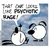## Going on a family trip June 27, 2019 by Cloudy176 I wasn't very active on the wiki recently, but I'm going to be even more inactive because I'll be on a trip to Canada with my family for the next 8~9 days or so. See you later! Read more > •## A hopefully powerful new system June 27, 2019 by Mh314159 Please comment on my latest attempt to climb toward TREE(3) as a personal intellectual challenge. Conceptually, here's how this works. The base function is successorship. Then there is a line of bracket-enclosed numbers or comma-separated strings of numbers. The last two brackets expressions and the "rank" and "argument". All others to their left are "orders". There are rules that recurse longer and more complex expressions down to simpler expressions. A number to the left of a backslash indicates the cycle of the expressions to its right and there is a powerful cycle factorial rule. In order from most complex to least complex, expressions recurse in the following order of rule sets: c > o > ss > sn > ns > nn > base. I think this generates… Read more > •## The T Array Function Part 5: Arbitrary Comma T Function (ACT) June 25, 2019 by SuperSpruce Here is the T array function, which now includes Arbitrary Comma arrays. This should reach ψ(Ωω). Attempt 3 at definition (fixes the notation getting stuck at ω^ω) A string is any expression in the outer brackets that is a number, comma, dimensional separator, or bracket with any subscript. An entry is a integer greater than or equal to 0. # , #' , % , and %' are any strings (can be empty) @ is any non-empty string.$ is any number of opening brackets (can be 0 opening brackets)

Entry a is at Layer x, where x is the amount of curly braces an entry is enclosed by. The base layer is x=0.

Parentheses disambiguate certain expressions; they do not mean a different array altogether.

Rules: [APPLY IF POSSIBLE BEFORE STARTING SCANNING PROCESS]

1. Base Rule:…

•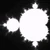## Tungster's Repeated Extension (Tungsten Extension)

June 24, 2019 by Tungster24

Note: Not done yet! I will expand this.

Mods: If you decide to add to the wiki remove this part and the possibilities section.

Tungster's Repeated Expansion or Tungsten Extension is a function repetition made by Tungster24 . It focuses on making a function grow by a simple systematic method.

Stages are the stages of the function.

Say x is a number. You want to apply f(x) over and over again for y times. that would be: %[x,f(x),y]. It's usable with any function. so %[2,x^2,2] = 16.

You can appy a function in a function. This gives us Stage 2

Say you had %[x,f(x),%[x,f(x),y]]. Or maybe 3,4 or 5 TE's Inside another. That could be shortened with a fourth character that states the number of repeats. If no repeats then we write in Stage 1.

Example: %[2,x…

•## I can't put a title to what just happened...

So, things on here may have gotten quiet, but oh boy, do I have news for you...

Jonathan Bowers joined the Googology Discord.

Yes, THE Jonathan Bowers. The man, the myth, the legend. Arguably the main founder of modern googology. He joined the Discord a while back, but didn't post until a few hours ago. But it's definitely him that's for sure.

No, I don't know how he found the server. No, I didn't belivee it either. But it has happened. Jonathan Bowers himself seems to have stumbled onto the rest of this community.

That's all for tonight, folks.

•## A computable function

June 23, 2019 by JHeroJr

My new function is the Fast-Growing Hierarchy Maximum function which is defined as FGHM(n) = the biggest finite number that can be represented with n symbols in the language of the Fast-Growing Hierarchy (a digit counts as a symbol). The language of the fast-growing hierarchy is fully defined here)

If no finite number can be made with n Fast-Growing Hierarchy symbols, then the return value is 0. Wow, it turns out that despite my thoughts, the FGH is uncomputable! I wish I could change this blog post's title...

I give up on this project. The link doesn't work.

•## Can Someone Tell Me How To Create An Uncomputable Function That Is Not Naive?

June 23, 2019 by JHeroJr

I really wanna make an uncomputable function to play around with. I know all about Turing machines and about what makes an uncomputable function uncomputable, but all the ideas are taken by Rayo's function, the Xi function, and the busy beaver function. And if a function were defined "f(n) = the smallest number not computable to a turing machine with a level-n oracle.", wouldn't all the values be undefined?

Pleeeeeaaaaase tell me how to make an uncomputable function!!!!!

•In BM4, (0,0,0)(1,1,1)(2,1,0)(1,1,1) expands to (0,0,0)(1,1,1)(2,1,0)(1,1,0)(2,2,1)(3,2,0)(2,2,0)(3,3,1)(4,3,0)(3,3,0)(4,4,1)(5,4,0)... not (0,0,0)(1,1,1)(2,1,0)(1,1,0)(2,2,1)(3,1,0)(2,2,0)(3,3,1)(4,1,0)(3,3,0)(4,4,1)(5,1,0)...,and this is known to make the analysis of BM4 very difficult past $$\psi(\Omega_\omega\times\Omega+\Omega_\omega)$$ in UNOCF. In BM4, if a standard matrix A does not contain (1,0) and $$A=\psi(C)$$ in UNOCF, A(1,1) is known to correspond to $$\psi(C+\Omega)$$ in UNOCF. However, even if a standard matrix A does not contain (1,0,0) and (1,1,0) and $$A=\psi(C)$$, A(1,1,1) does not necessarily correspond to $$\psi(C+\Omega_\omega)$$. Because this makes the analysis a lot harder, many people have tried to make a version …